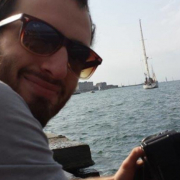## 15/04/2021, Thursday, 15:00–16:00 Europe/Lisbon — OnlineRiccardo Scala, Università degli Studi di Siena
Nonlocality features of the area functional and the Plateau problem

We briefly discuss the definition of relaxation of the area functional. The relaxed area functional, denoted by $A$, extends the classical area functional, which, for any "regular" map $v:U\subset \mathbb{R}^n\rightarrow \mathbb{R}^N$ evaluates the $n$-dimensional area of its graph over $U$. The problem of determining the domain and the expression of $A$ is open in codimension greater than 1. Specifically, this relaxed functional turns out to be nonlocal and cannot be expressed by an integral formula. We discuss how it is related to classical and nonclassical versions of the Plateau problem. As a main example, we try to understand what is the relaxed graph of the function $x/|x|$, a question that surprisingly remained open for decades.